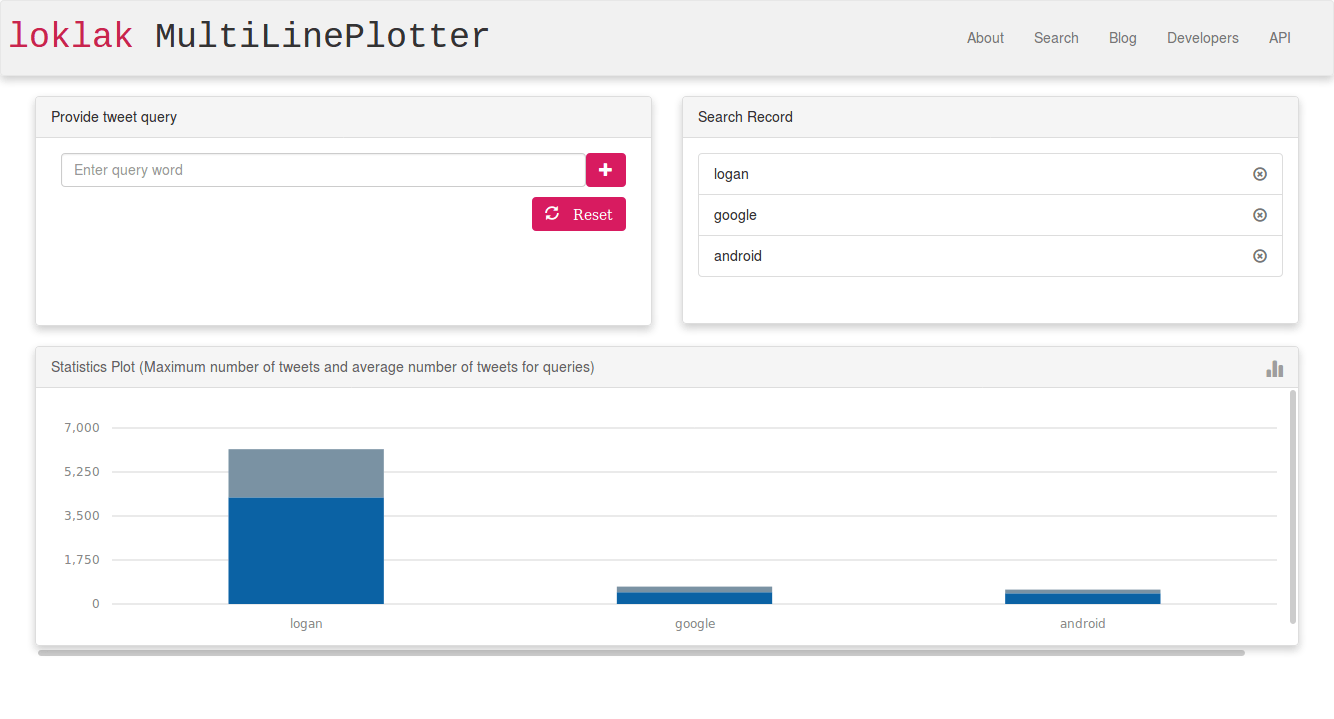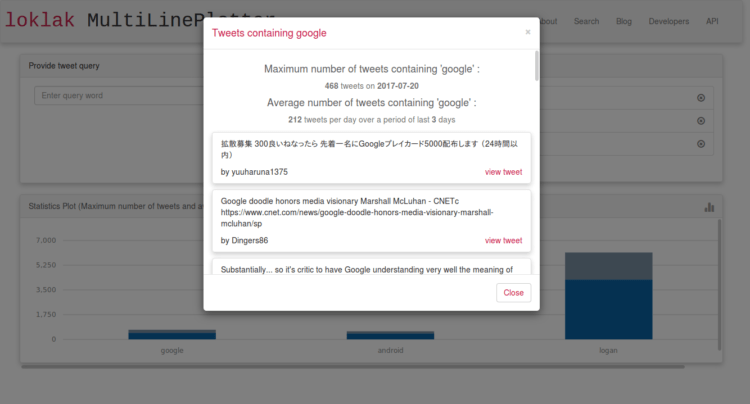# Visualising Tweet Statistics in MultiLinePlotter App for Loklak Apps

MultiLinePlotter app is now a part of Loklak apps site. This app can be used to compare aggregations of tweets containing a particular query word and visualise the data for better comparison. Recently there has been a new addition to the app. A feature for showing tweet statistics like the maximum number of tweets (along with date) containing the given query word and the average number of tweets over a period of time. Such statistics is visualised for all the query words for better comparison.

Related issue: https://github.com/fossasia/apps.loklak.org/issues/236

## Obtaining Maximum number of tweets and average number of tweets

Before visualising the statistics we need to obtain them. For this we simply need to process the aggregations returned by the Loklak API. Let us start with maximum number of tweets containing the given keyword. What we actually require is what is the maximum number of tweets that were posted and contained the user given keyword and on which date the number was maximum. For this we can use a function which will iterate over all the aggregations and return the largest along with date.

```\$scope.getMaxTweetNumAndDate = function(aggregations) {
var maxTweetDate = null;
var maxTweetNum = -1;

for (date in aggregations) {
if (aggregations[date] > maxTweetNum) {
maxTweetNum = aggregations[date];
maxTweetDate = date;
}
}

return {date: maxTweetDate, count: maxTweetNum};
}

```

The above function maintains two variables, one for maximum number of tweets and another for date. We iterate over all the aggregations and for each aggregation we compare the number of tweets with the value stored in the maxTweetNum variable. If the current value is more than the value stored in that variable then we simply update it and keep track of the date. Finally we return an object containing both maximum number of tweets and the corresponding date.Next we need to obtain average number of tweets. We can do this by summing up all the tweet frequencies and dividing it by number of aggregations.

```\$scope.getAverageTweetNum = function(aggregations) {
var avg = 0;
var sum = 0;

for (date in aggregations) {
sum += aggregations[date];
}

return parseInt(sum / Object.keys(aggregations).length);
}

```

The above function calculates average number of tweets in the way mentioned before the snippet.

Next for every tweet we need to store these values in a format which can easily be understood by morris.js. For this we use a list and store the statistics values for individual query words as objects and later pass it as a parameter to morris.

```var maxStat = \$scope.getMaxTweetNumAndDate(aggregations);
var avg = \$scope.getAverageTweetNum(aggregations);

\$scope.tweetStat.push({
tweet: \$scope.tweet,
maxTweetCount: maxStat.count,
maxTweetOn: maxStat.date,
averageTweetsPerDay: avg,
aggregationsLength: Object.keys(aggregations).length
});

```

We maintain a list called tweetStat and the list contains objects which stores the query word and the corresponding values.

Apart from plotting these statistics, the app also displays the statistics when user clicks on an individual treat present in the search record section. For this we filter tweetStat list mentioned above and get the required object corresponding to the query word the user selected bind it to angular scope. Next we display it using HTML.

```<div class="tweet-stat max-tweet">
<div class="stat-label"> <h4>Maximum number of tweets containing '{{modalHeading}}' :</h4></div>
<div class="stat-value"> <strong>{{selectedTweetStat.maxTweetCount}}</strong> tweets on
<strong>{{selectedTweetStat.maxTweetOn}}</strong>
</div>
</div>

```Finally we need to plot the statistics. For this we use a function called plotStatGraph dedicated only for plotting statistics graph. We pass the tweetStat list as a parameter to morris and configure all the other parameters.

```\$scope.plotStatGraph = function() {
\$scope.plotStat = new Morris.Bar({
element: 'graph',
data: \$scope.tweetStat,
xkey: 'tweet',
ykeys: ['maxTweetCount', 'averageTweetsPerDay'],
labels: ['Maximum no. of tweets : ', 'Average no. of tweets/day'],
parseTime: false,
hideHover: 'auto',
resize: true,
stacked: true,
barSizeRatio: 0.40
});
}

```

But now we have two graphs. One for showing variations in aggregation and the other for showing statistics. How do we manage them? Somehow we need to show them in the same page as this is a single page app. Also we need to avoid vertical scrolling as it would degrade both UI and UX. So we need to implement a switching mechanism. The user should be able to switch between the two graph views as per their wish. How to achieve that? Well, for this we maintain a global variable which will keep track of the current plot type. If the current graph type is aggregations then we call the function to plot aggregations otherwise we call the above mentioned function to plot statistics.

```\$scope.plotData = function() {
\$(".plot-data").html("");
if (\$scope.currentGraphType === "aggregations") {
\$scope.plotAggregationGraph();
} else {
\$scope.plotStatGraph();
}
}

```

Lastly we integrate this state variable (currentGraphType) with the UI so that users can easily toggle between graph views with just a click.

```<div class="switch" ng-click="toggle()">
<span ng-if="queryRecords.length !== 0" class="glyphicon glyphicon-stats"></span>
</div>

```## Important resources

#### Deepjyoti Mondal

A web and mobile application developer and an enthusiastic learner. I like trying out new technologies. JavaScript and Python are my favorites